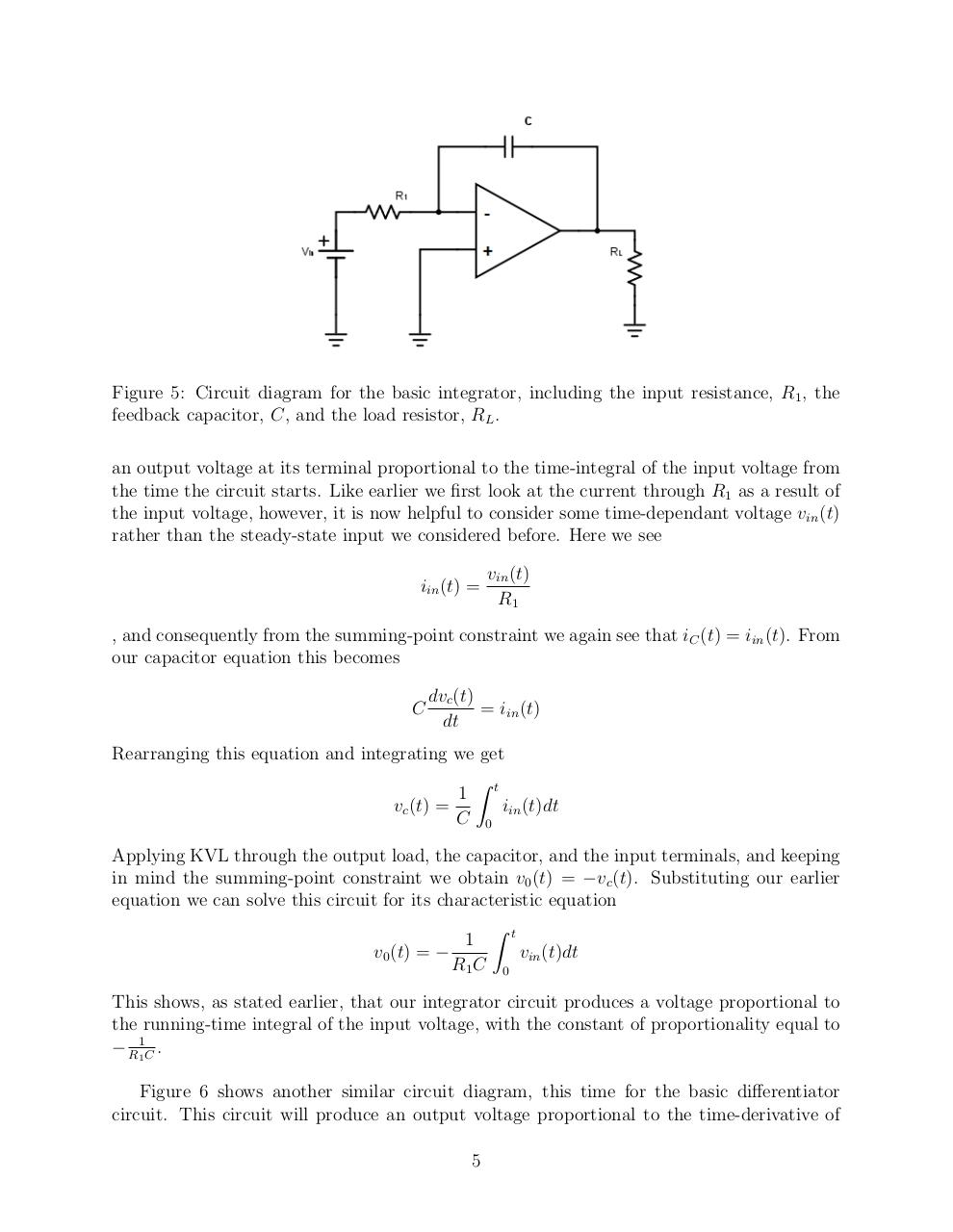# Op Amps.pdfPage 1 2 3 4 5 6 7 8 9 10 11 12 13

#### Text preview

Figure 5: Circuit diagram for the basic integrator, including the input resistance, R1 , the
feedback capacitor, C, and the load resistor, RL .
an output voltage at its terminal proportional to the time-integral of the input voltage from
the time the circuit starts. Like earlier we first look at the current through R1 as a result of
the input voltage, however, it is now helpful to consider some time-dependant voltage vin (t)
rather than the steady-state input we considered before. Here we see
iin (t) =

vin (t)
R1

, and consequently from the summing-point constraint we again see that iC (t) = iin (t). From
our capacitor equation this becomes
C

dvc (t)
= iin (t)
dt

Rearranging this equation and integrating we get
Z
1 t
vc (t) =
iin (t)dt
C 0
Applying KVL through the output load, the capacitor, and the input terminals, and keeping
in mind the summing-point constraint we obtain v0 (t) = −vc (t). Substituting our earlier
equation we can solve this circuit for its characteristic equation
Z t
1
v0 (t) = −
vin (t)dt
R1 C 0
This shows, as stated earlier, that our integrator circuit produces a voltage proportional to
the running-time integral of the input voltage, with the constant of proportionality equal to
− R11C .
Figure 6 shows another similar circuit diagram, this time for the basic differentiator
circuit. This circuit will produce an output voltage proportional to the time-derivative of
5